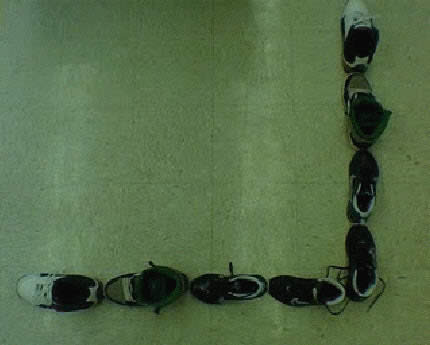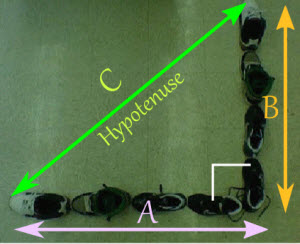# Activity: Pythagoras' Theorem

Got a Lot of Shoes? Let's Learn Pythagoras' Theorem!

What you'll need:

• shoes
• ruler
• pen and paper

1st: Gather up as many shoes as you can.

2nd: Since Pythagoras' Theorem only works for 90 degree triangles, line your shoes up to form the letter L, like this:Or this:3rd: Label one line of shoes A, and the other line of shoes B (you could call them "legs" of a triangle!)4th: Measure each line of shoes with your measuring device and record them on your paper

 A = B =

Now we have enough information to solve the distance from the tip of one line of shoes to the tip of the other line of shoes. We call this line the hypotenuse

5th: Using Pythagoras' Theorem A2 + B2 = C2 solve for the distance of C, our hypotenuse.

C = √( A2 + B2 )

6th: Plug in your recorded information for A and B and solve for C. Hint: make sure your using the same units like inches or cm's. Record your answer below

 C (by calculation) =

7th: After recording your data, use your measuring device to measure the hypotenuse (the distance from the tip of one line of shoes to the tip of the other line of shoes). Did you get the same answer?

 C (by measurement) =